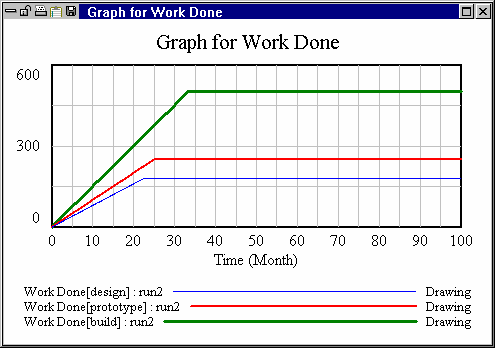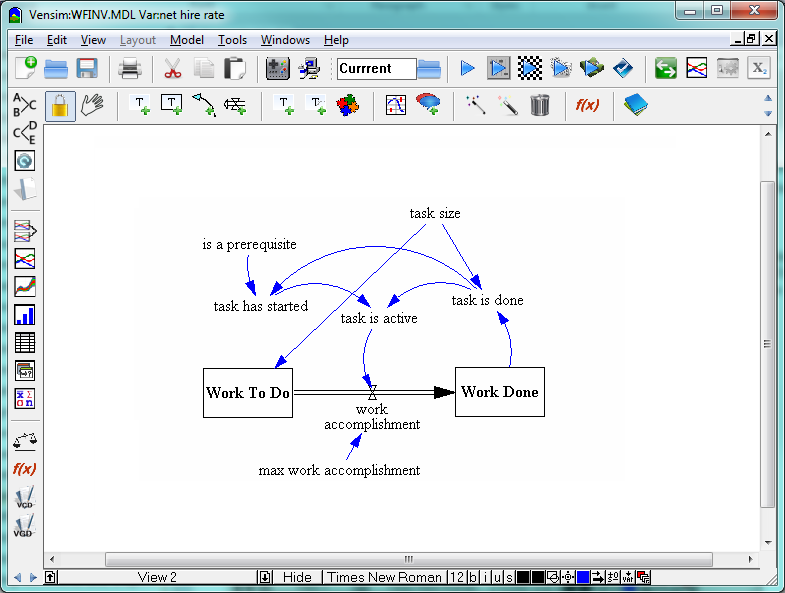# Vensim Help

 Limits to Rabbit Growth

The population model of Chapter 6 is extended here to include the consequences of population growth in an environment with a limited carrying capacity.  The unchecked growth of the previous model (pop.mdl) is replaced by growth which is limited by the size of the population relative to the carrying capacity of the local environment (rabbit.mdl).

The value of the variable deaths in pop.mdl is directly proportional to the size of the rabbit population.  In effect, we see a relationship between Population and deaths that is linear:This does not mean that deaths will increase linearly over time.  The linearity means deaths will grow in the same manner as Population (if Population grows exponentially, so will deaths).

We could make a Lookup table (as above) that expresses this linear relationship, but it is easier just to use a constant (that has the same value as the slope of the Lookup) multiplied by Population.  In fact, the model developed in Chapter 6 uses Population/average lifetime so that the slope of the straight line would be 1/average lifetime.  This formulation was chosen because it is much easier to understand what average lifetime is (and what a change in it means) than to understand the shape and slope of a curve.

What we want to do in this model is have deaths increase more quickly than Population as population grows to a large size.   This happens because higher populations are nearer resource limits (such as food) and therefore rabbits will, on average, die more quickly.  We are looking for a function such as:You can develop such a function using Lookups, and that is what you will do next.  Before that happens, however, it is important normalize the input and output of the Lookup.